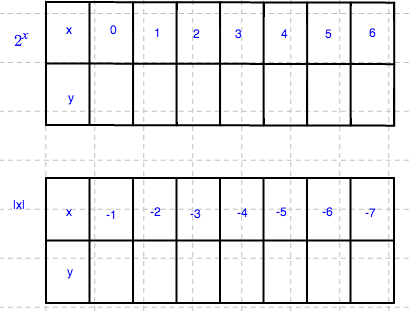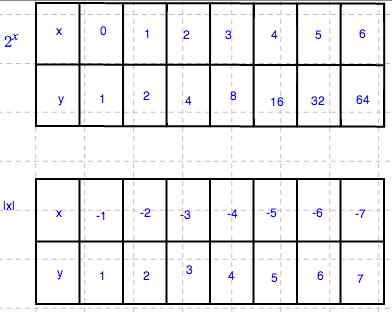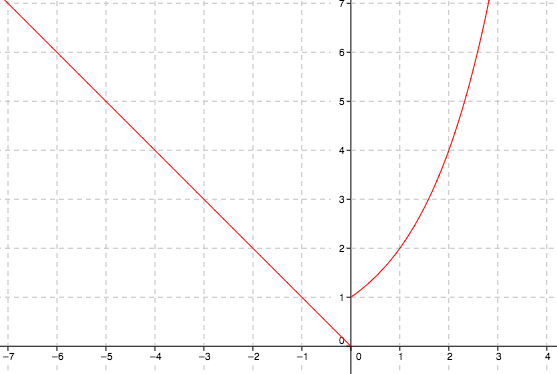### Home > CCA2 > Chapter C > Lesson C.1.3 > ProblemC-59

C-59.

Graph the piecewise function $f ( x ) = \left\{ \begin{array} { l l } { 2 ^ { x } } & { \text { if } x \geq 0 } \\ { | x | } & { \text { if } x < 0 } \end{array} \right.$.

Start by making a table of points for each of the two parts of the function.